# 题目描述（困难难度）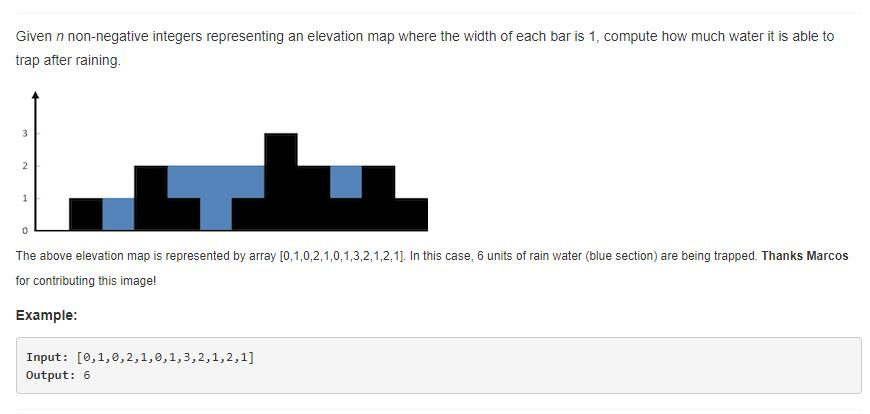# 解法一 按行求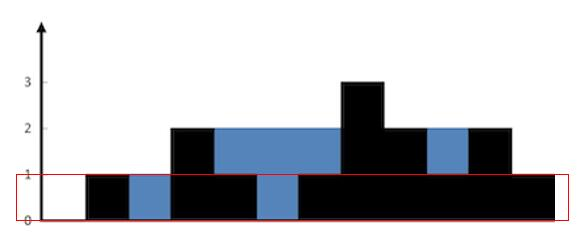temp 初始化为 0 ，ans = 0

height [ 0 ] 等于 0 < 1，不更新。

height [ 1 ] 等于 1 >= 1，开始更新 temp。

height [ 2 ] 等于 0 < 1， temp = temp + 1 = 1。

height [ 3 ] 等于 2 >= 1， ans = ans + temp = 1，temp = 0。

height [ 4 ] 等于 1 >= 1，ans = ans + temp = 1，temp = 0。

height [ 5 ] 等于 0 < 1， temp = temp + 1 = 1。

height [ 6 ] 等于 1 >= 1，ans = ans + temp = 2，temp = 0。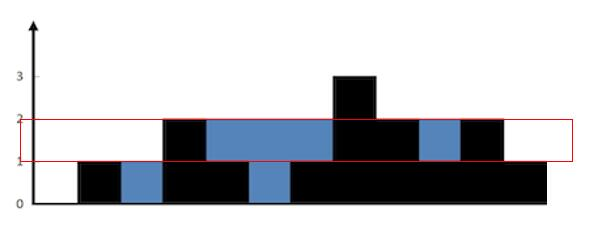temp 初始化为 0 ，ans 此时等于 2。

height [ 0 ] 等于 0 < 2，不更新。

height [ 1 ] 等于 1 < 2，不更新。

height [ 2 ] 等于 0 < 2， 不更新。

height [ 3 ] 等于 2 >= 2， 开始更新

height [ 4 ] 等于 1 < 2，temp = temp + 1 = 1。

height [ 5 ] 等于 0 < 2， temp = temp + 1 = 2。

height [ 6 ] 等于 1 < 2， temp = temp + 1 = 3。

height [ 7 ] 等于 3 >= 2， ans = ans + temp = 5，temp = 0。

height [ 8 ] 等于 2 >= 2， ans = ans + temp = 3，temp = 0。

height [ 9 ] 等于 1 < 2， temp = temp + 1 = 1。

height [ 10 ] 等于 2 >= 2， ans = ans + temp = 6，temp = 0。

height [ 11 ] 等于 1 < 2， temp = temp + 1 = 1。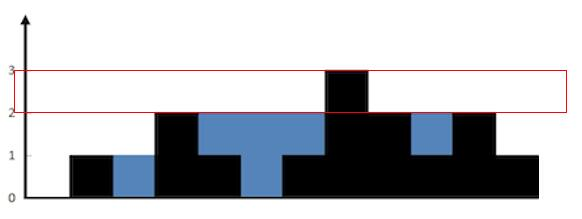public int trap(int[] height) {
int sum = 0;
int max = getMax(height);//找到最大的高度，以便遍历。
for (int i = 1; i <= max; i++) {
boolean isStart = false; //标记是否开始更新 temp
int temp_sum = 0;
for (int j = 0; j < height.length; j++) {
if (isStart && height[j] < i) {
temp_sum++;
}
if (height[j] >= i) {
sum = sum + temp_sum;
temp_sum = 0;
isStart = true;
}
}
}
return sum;
}

private int getMax(int[] height) {
int max = 0;
for (int i = 0; i < height.length; i++) {
if (height[i] > max) {
max = height[i];
}
}
return max;
}


# 解法二 按列求

• 较矮的墙的高度大于当前列的墙的高度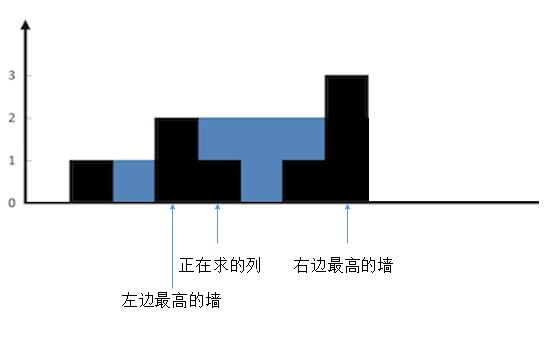把正在求的列左边最高的墙和右边最高的墙确定后，然后为了方便理解，我们把无关的墙去掉。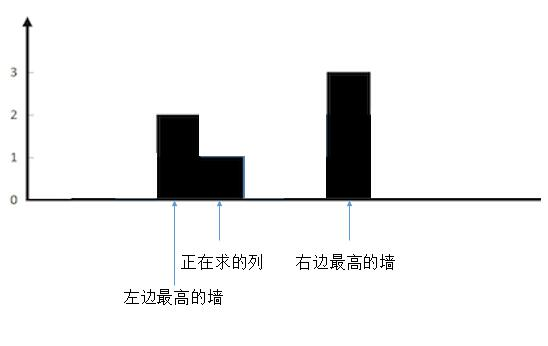这样就很清楚了，现在想象一下，往两边最高的墙之间注水。正在求的列会有多少水？

很明显，较矮的一边，也就是左边的墙的高度，减去当前列的高度就可以了，也就是 2 - 1 = 1，可以存一个单位的水。

• 较矮的墙的高度小于当前列的墙的高度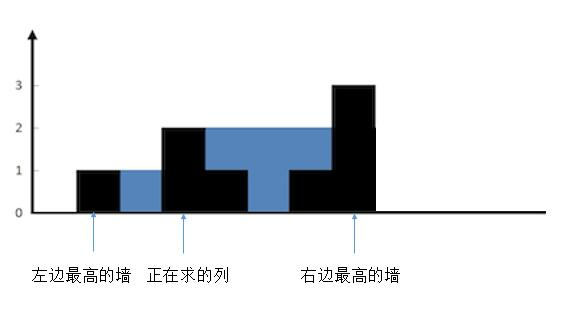同样的，我们把其他无关的列去掉。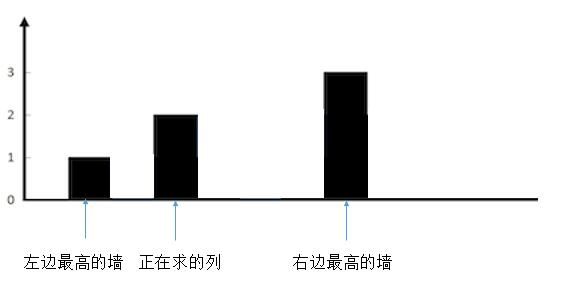想象下，往两边最高的墙之间注水。正在求的列会有多少水？

正在求的列不会有水，因为它大于了两边较矮的墙。

• 较矮的墙的高度等于当前列的墙的高度。

和上一种情况是一样的，不会有水。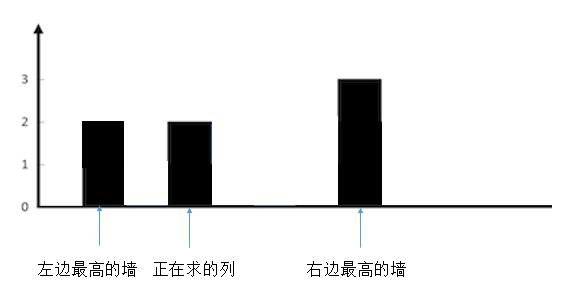public int trap(int[] height) {
int sum = 0;
//最两端的列不用考虑，因为一定不会有水。所以下标从 1 到 length - 2
for (int i = 1; i < height.length - 1; i++) {
int max_left = 0;
//找出左边最高
for (int j = i - 1; j >= 0; j--) {
if (height[j] > max_left) {
max_left = height[j];
}
}
int max_right = 0;
//找出右边最高
for (int j = i + 1; j < height.length; j++) {
if (height[j] > max_right) {
max_right = height[j];
}
}
//找出两端较小的
int min = Math.min(max_left, max_right);
//只有较小的一段大于当前列的高度才会有水，其他情况不会有水
if (min > height[i]) {
sum = sum + (min - height[i]);
}
}
return sum;
}


# 解法三 动态规划

max_left [ i ] = Max ( max_left [ i - 1] , height [ i - 1]) 。它前边的墙的左边的最高高度和它前边的墙的高度选一个较大的，就是当前列左边最高的墙了。

max_right[ i ] = Max ( max_right[ i + 1] , height [ i + 1]) 。它后边的墙的右边的最高高度和它后边的墙的高度选一个较大的，就是当前列右边最高的墙了。

public int trap(int[] height) {
int sum = 0;
int[] max_left = new int[height.length];
int[] max_right = new int[height.length];

for (int i = 1; i < height.length - 1; i++) {
max_left[i] = Math.max(max_left[i - 1], height[i - 1]);
}
for (int i = height.length - 2; i >= 0; i--) {
max_right[i] = Math.max(max_right[i + 1], height[i + 1]);
}
for (int i = 1; i < height.length - 1; i++) {
int min = Math.min(max_left[i], max_right[i]);
if (min > height[i]) {
sum = sum + (min - height[i]);
}
}
return sum;
}


# 解法四 双指针

public int trap(int[] height) {
int sum = 0;
int max_left = 0;
int[] max_right = new int[height.length];
for (int i = height.length - 2; i >= 0; i--) {
max_right[i] = Math.max(max_right[i + 1], height[i + 1]);
}
for (int i = 1; i < height.length - 1; i++) {
max_left = Math.max(max_left, height[i - 1]);
int min = Math.min(max_left, max_right[i]);
if (min > height[i]) {
sum = sum + (min - height[i]);
}
}
return sum;
}


max_left = Math.max(max_left, height[i - 1]);


height [ left - 1] 是可能成为 max_left 的变量， 同理，height [ right + 1 ] 是可能成为 right_max 的变量。

public int trap(int[] height) {
int sum = 0;
int max_left = 0;
int max_right = 0;
int left = 1;
int right = height.length - 2; // 加右指针进去
for (int i = 1; i < height.length - 1; i++) {
//从左到右更
if (height[left - 1] < height[right + 1]) {
max_left = Math.max(max_left, height[left - 1]);
int min = max_left;
if (min > height[left]) {
sum = sum + (min - height[left]);
}
left++;
//从右到左更
} else {
max_right = Math.max(max_right, height[right + 1]);
int min = max_right;
if (min > height[right]) {
sum = sum + (min - height[right]);
}
right--;
}
}
return sum;
}


# 解法五 栈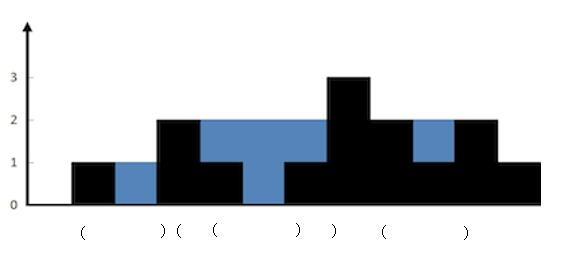1. 当前高度小于等于栈顶高度，入栈，指针后移。

2. 当前高度大于栈顶高度，出栈，计算出当前墙和栈顶的墙之间水的多少，然后计算当前的高度和新栈的高度的关系，重复第 2 步。直到当前墙的高度不大于栈顶高度或者栈空，然后把当前墙入栈，指针后移。

• 首先将 height [ 0 ] 入栈。然后 current 指向的高度大于栈顶高度，所以把栈顶 height [ 0 ] 出栈，然后栈空了，再把 height [ 1 ] 入栈。current 后移。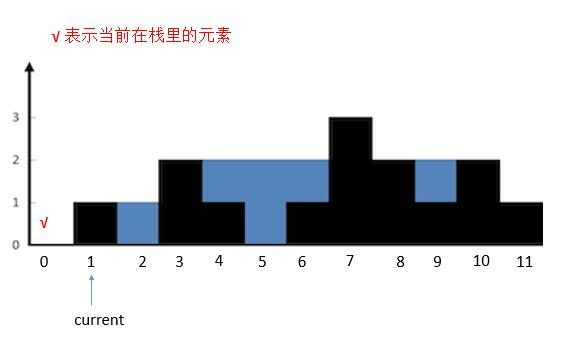• 然后 current 指向的高度小于栈顶高度，height [ 2 ] 入栈，current 后移。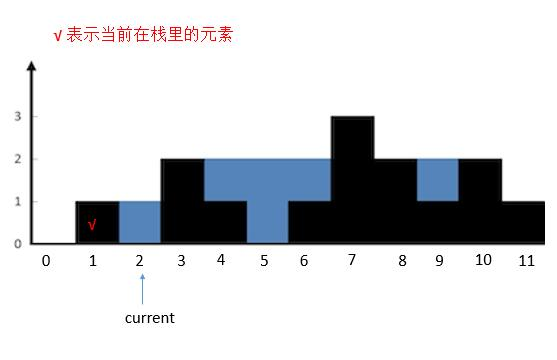• 然后 current 指向的高度大于栈顶高度，栈顶 height [ 2 ] 出栈。计算 height [ 3 ] 和新的栈顶之间的水。计算完之后继续判断 current 和新的栈顶的关系。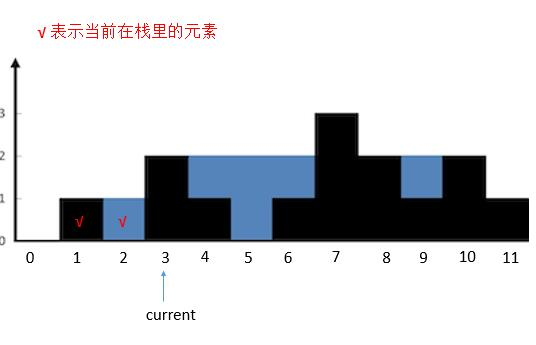• current 指向的高度大于栈顶高度，栈顶 height [ 1 ] 出栈，栈空。所以把 height [ 3 ] 入栈。 currtent 后移。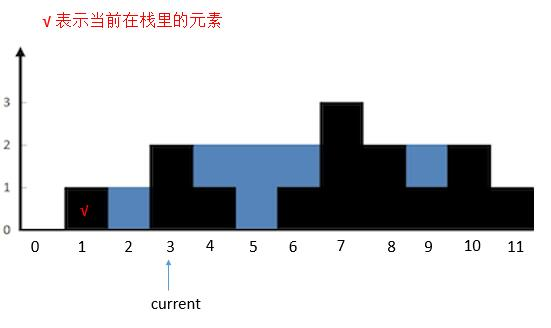• 然后 current 指向的高度小于栈顶 height [ 3 ] 的高度，height [ 4 ] 入栈。current 后移。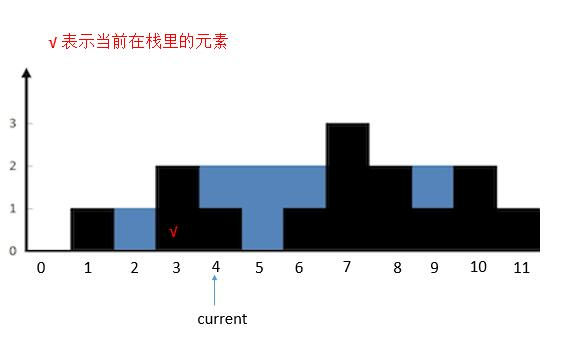• 然后 current 指向的高度小于栈顶 height [ 4 ] 的高度，height [ 5 ] 入栈。current 后移。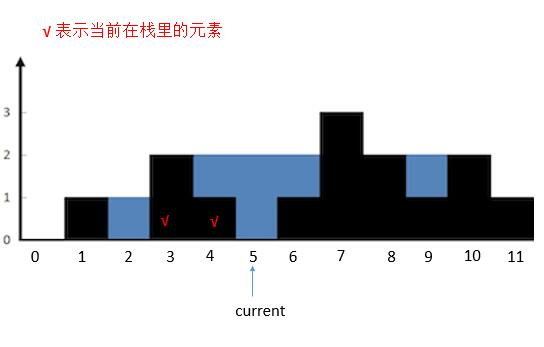• 然后 current 指向的高度大于栈顶 height [ 5 ] 的高度，将栈顶 height [ 5 ] 出栈，然后计算 current 指向的墙和新栈顶 height [ 4 ] 之间的水。计算完之后继续判断 current 的指向和新栈顶的关系。此时 height [ 6 ] 不大于栈顶 height [ 4 ] ，所以将 height [ 6 ] 入栈。 current 后移。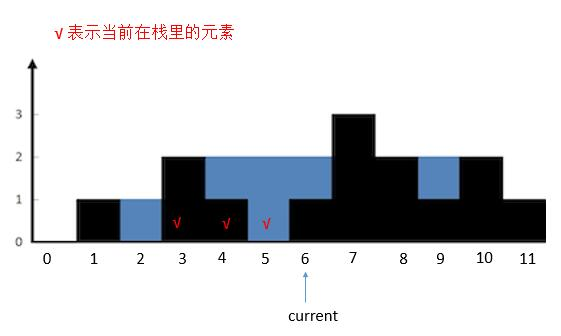• 然后 current 指向的高度大于栈顶高度，将栈顶 height [ 6 ] 出栈。计算和新的栈顶 height [ 4 ] 组成两个边界中的水。然后判断 current 和新的栈顶 height [ 4 ] 的关系，依旧是大于，所以把 height [ 4 ] 出栈。计算current 和 新的栈顶 height [ 3 ] 之间的水。然后判断 current 和新的栈顶 height [ 3 ] 的关系，依旧是大于，所以把 height [ 3 ] 出栈，栈空。将 current 指向的 height [ 7 ] 入栈。current 后移。

其实不停的出栈，可以看做是在找与 7 匹配的墙，也就是 3 。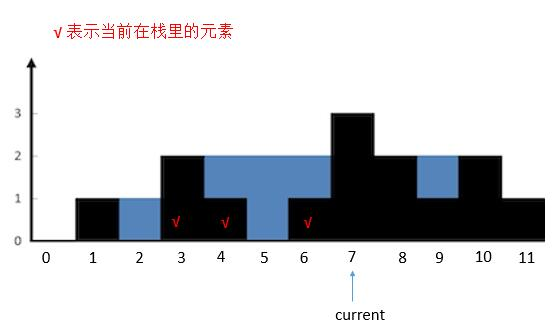public int trap6(int[] height) {
int sum = 0;
Stack<Integer> stack = new Stack<>();
int current = 0;
while (current < height.length) {
//如果栈不空并且当前指向的高度大于栈顶高度就一直循环
while (!stack.empty() && height[current] > height[stack.peek()]) {
int h = height[stack.peek()]; //取出要出栈的元素
stack.pop(); //出栈
if (stack.empty()) { // 栈空就出去
break;
}
int distance = current - stack.peek() - 1; //两堵墙之前的距离。
int min = Math.min(height[stack.peek()], height[current]);
sum = sum + distance * (min - h);
}
stack.push(current); //当前指向的墙入栈
current++; //指针后移
}
return sum;
}